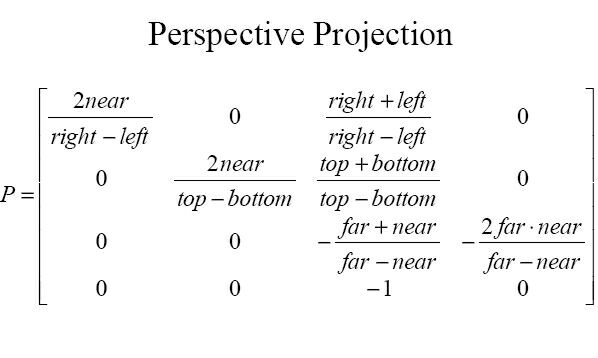Camera space --> screen space, ie. 'perspective projection'
So you have successfully transformed vertices from object space to world space to camera space using matrix multiplications. Now what?

What you have now is a coordinate system where the (0,0,0) is right in the camera center, and +Z sticks out of the camera along the 'gaze' direction. Your vertices are expressed in such a coordinate system (camera space).

We want to limit what the camera sees by only considering verts that lie totally inside a view frustum (truncated square pyramid). This frustum can be expressed using pairs of distances along the XYZ axes, ie left, right, top, bottom, near and far.

The perspective transform matrix (in the slide following the 'Pseudodepth' one) correctly encodes a perspective transformation where the point (left,bottom,-near) will turn into (-1,-1,-1) and (right,top,-far) will map to (1,1,1).

If you do the multiplication by hand (use [left,bottom,-near,1] and [right,top,-far,1] for the point, and post-multiply the point with the matrix, ie. do matrix*point) you will see that you get (-near,-near,-near,near) and (near,near,near,near) respectively. To get (-1,-1,-1) and (1,1,1), you'd have to divide the resulting (x,y,z) each by w, the fourth ("homogeneous") coordinate. For our points 'w' happens to be 'near', so we'd get (-1,-1,-1) and (1,1,1) as desired.

What all this boils down to is this - to go from a point in camera space to "NDC" (where x,y,z are all within the -1..1 range), first create the 4x4 perspective transformation matrix shown below (taken from the lecture):Next, multiply the matrix by the cam-space point (which is a homogeneous 4D point with a '1' at the end). Take the resulting point (call it x,y,z,w and divide x,y and z by w). The result will be in -1..1 NDC space (if it isn't, that simply means that near, far, top, bottom, left and right are not 'big' enough to frame the object, and you can keep increasing their size till all vertices lie in -1..1).

So THAT is the key - if multiplication with the perspective matrix gives you (x',y'z',h), you'd turn that into 3D coordinates (x'' = x'/h, y'' = y'/h, z'' = z'/h).

We can throw away z'' since (x'',y'') alone captures the result of the projection on a flat plane. If our vertex lies inside the view volume, x'' and y'' will each be between -1.0 and 1.0. You can convert these to integer coords using (0.5*(1+x''), 0.5*(1+y")) to first convert to the 0 to 1 range, and multiplying the result by (xres-1) and (yres-1) and truncating. That will give you an integer pixel coordinate that lies between (0,0) and (xres-1,yres-1).

In other words once you get all verts to be in -1..1, take just the x and y of each and turn them into pixel coordinates:
pixel_x = (NDC_x+1)*0.5*(xres-1)
pixel_y = (NDC_y+1)*0.5*(yres-1)

If you do the above steps for all vertex pairs that define edges in your polymesh, you can draw digital lines to connect those pairs, and voila - a wireframe result :)